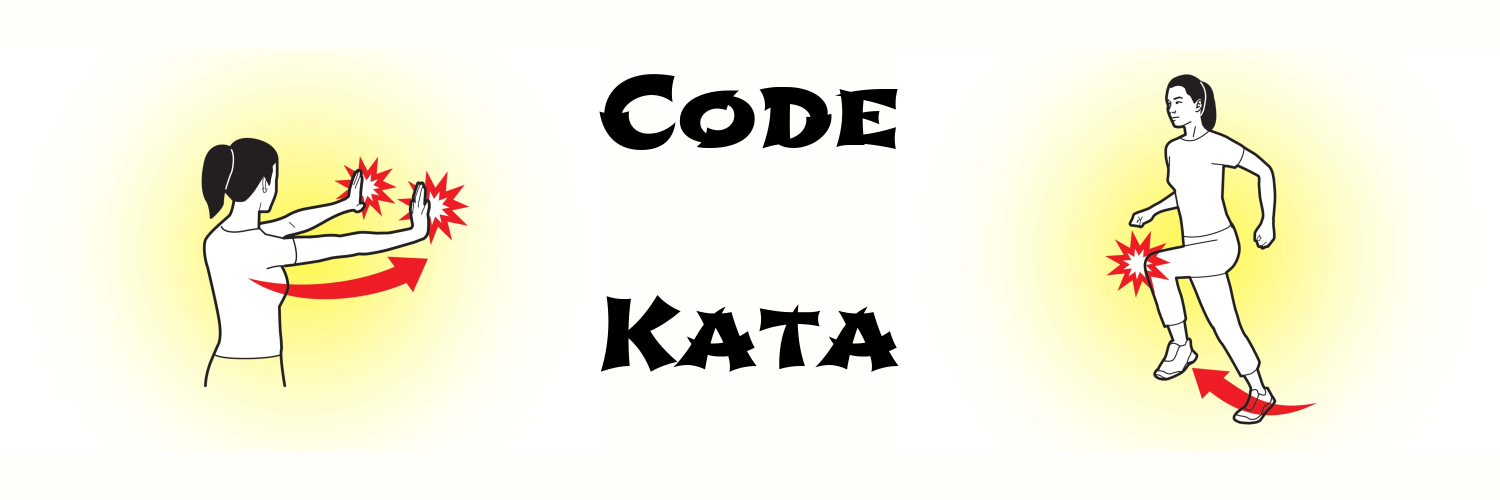# programming kata

## Programming Kata Day 8Problem Write a function steps, that takes a sequence and makes a deeply nested structure from it: (steps [1 2 3 4]) ;=> [1 [2 [3 [4 []]]]] Solution 1 (defn steps [s] (if (seq s) [(first s) (steps (rest s))] [])) Solution 2 Lazy version: (defn lz-steps [s] (lazy-seq (if (seq s) [(first s) (lz-steps (rest s))] []))) Note To see the difference, call the function like this:

## Programming Kata Day 7Problem Suppose a binary tree structure looks like this: {:val value :L <left-node> :R <right-node>} Write a function to balance insert node into the tree. Solution (defn insert [tree x] (cond (nil? tree) {:val x :L nil :R nil} (< x (:val tree)) (assoc tree :L (insert (:L tree) x)) :else (assoc tree :R (insert (:R tree) x)))) Note Here is the function to traverse the tree: (defn traverse [tree] (when tree (concat (traverse (:L tree)) [(:val tree)] (traverse (:R tree))))) Here is a helper function to create a tree.

## Programming Kata Day 6